Courses

# Thermodynamic Relations Physics Notes | EduRev

## Physics : Thermodynamic Relations Physics Notes | EduRev

The document Thermodynamic Relations Physics Notes | EduRev is a part of the Physics Course Basic Physics for IIT JAM.
All you need of Physics at this link: Physics

Work of expansion and contraction:

The first task in carrying out the above program is to calculate the amount of work done by a single pure substance when it expands at constant temperature. Unlike the case of a chemical reaction, where the volume can change at constant temperature and pressure because of the liberation of gas, the volume of a single pure substance placed in a cylinder cannot change unless either the pressure or the temperature changes. To calculate the work, suppose that a piston moves by an infinitesimal amount dx. Because pressure is force per unit area, the total restraining force exerted by the piston on the gas is PA, where A is the cross-sectional area of the piston. Thus, the incremental amount of work done is dW = PA dx.
However, A dx can also be identified as the incremental change in the volume (dV) swept out by the head of the piston as it moves. The result is the basic equation dW = P dV for the incremental work done by a gas when it expands. For a finite change from an initial volume Vi to a final volume Vf, the total work done is given by the integral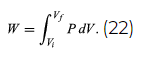Because P in general changes as the volume V changes, this integral cannot be calculated until P is specified as a function of V; in other words, the path for the process must be specified. This gives precise meaning to the concept that dW is not an exact differential.

Heat capacity and specific heat:

As shown originally by Count Rumford, there is an equivalence between heat (measured in calories) and mechanical work (measured in joules) with a definite conversion factor between the two. The conversion factor, known as the mechanical equivalent of heat, is 1 calorie = 4.184 joules. (There are several slightly different definitions in use for the calorie. The calorie used by nutritionists is actually a kilocalorie.) In order to have a consistent set of units, both heat and work will be expressed in the same units of joules.
The amount of heat that a substance absorbs is connected to its temperature change via its molar specific heat c, defined to be the amount of heat required to change the temperature of 1 mole of the substance by 1 K. In other words, c is the constant of proportionality relating the heat absorbed (dQ) to the temperature change (dT) according to dQ = nc dT, where n is the number of moles. For example, it takes approximately 1 calorie of heat to increase the temperature of 1 gram of water by 1 K. Since there are 18 grams of water in 1 mole, the molar heat capacity of water is 18 calories per K, or about 75 joules per K. The total heat capacity C for n moles is defined by C = nc.
However, since dQ is not an exact differential, the heat absorbed is path-dependent and the path must be specified, especially for gases where the thermal expansion is significant. Two common ways of specifying the path are either the constant-pressure path or the constant-volume path. The two different kinds of specific heat are called cP and cV respectively, where the subscript denotes the quantity that is being held constant. It should not be surprising that cP is always greater than cV, because the substance must do work against the surrounding atmosphere as it expands upon heating at constant pressure but not at constant volume. In fact, this difference was used by the 19th-century German physicist Julius Robert von Mayer to estimate the mechanical equivalent of heat.

Heat capacity and internal energy:
The goal in defining heat capacity is to relate changes in the internal energy to measured changes in the variables that characterize the states of the system. For a system consisting of a single pure substance, the only kind of work it can do is atmospheric work, and so the first law reduces to
dU = dQ − P dV.  (28)

Suppose now that U is regarded as being a function U(TV) of the independent pair of variables T and V. The differential quantity dU can always be expanded in terms of its partial derivatives according to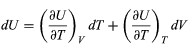(29)

where the subscripts denote the quantity being held constant when calculating derivatives. Substituting this equation into dU = dQ − P dV then yields the general expression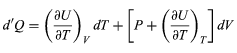(30)

for the path-dependent heat. The path can now be specified in terms of the independent variables T and V. For a temperature change at constant volume, dV = 0 and, by definition of heat capacity,
dQV = CV dT.         (31)
The above equation then gives immediately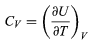(32)

for the heat capacity at constant volume, showing that the change in internal energy at constant volume is due entirely to the heat absorbed.
To find a corresponding expression for CP, one need only change the independent variables to T and P and substitute the expansion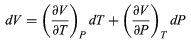(33)

for dV in equation and correspondingly for dU to obtain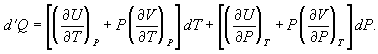(34)

For a temperature change at constant pressuredP = 0, and, by definition of heat capacity, dQ = CP dT, resulting in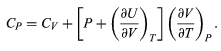(35)

The two additional terms beyond CV have a direct physical meaning. The term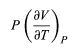Represents the additional atmospheric work that the system does as it undergoes thermal expansion at constant pressure, and the second term involving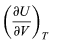represents the internal work that must be done to pull the system apart against the forces of attraction between the molecules of the substance (internal stickiness). Because there is no internal stickiness for an ideal gas, this term is zero, and, from the ideal gas law, the remaining partial derivative is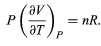(36)

With these substitutions the equation for CP becomes simply
CP = CV + nR    (37)
or
cP = cV + R    (38)

for the molar specific heats. For example, for a monoatomic ideal gas (such as helium), cV = 3R/2 and cP = 5R/2 to a good approximation. cVT represents the amount of translational kinetic energy possessed by the atoms of an ideal gas as they bounce around randomly inside their container. Diatomic molecules (such as oxygen) and polyatomic molecules (such as water) have additional rotational motions that also store thermal energy in their kinetic energy of rotation. Each additional degree of freedom contributes an additional amount R to cV. Because diatomic molecules can rotate about two axes and polyatomic molecules can rotate about three axes, the values of cV increase to 5R/2 and 3R respectively, and cP correspondingly increases to 7R/2 and 4R. (cV and cP increase still further at high temperatures because of vibrational degrees of freedom.) For a real gas such as water vapour, these values are only approximate, but they give the correct order of magnitude. For example, the correct values are cP = 37.468 joules per K (i.e., 4.5R) and cP − cV = 9.443 joules per K (i.e., 1.14R) for water vapour at 100 °C and 1 atmosphere pressure.

Offer running on EduRev: Apply code STAYHOME200 to get INR 200 off on our premium plan EduRev Infinity!

## Basic Physics for IIT JAM

77 videos|156 docs|94 tests

,

,

,

,

,

,

,

,

,

,

,

,

,

,

,

,

,

,

,

,

,

;